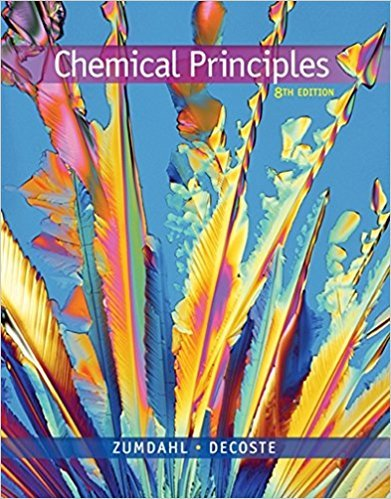×
Get Full Access to Chemical Principles - 8 Edition - Chapter 15 - Problem 15.84
Get Full Access to Chemical Principles - 8 Edition - Chapter 15 - Problem 15.84

×

# The reaction (CH3)3CBr 1 OH2 88n (CH3)3COH 1 Br2 in aISBN: 9781305581982 176

## Solution for problem 15.84 Chapter 15

Chemical Principles | 8th Edition

• Textbook Solutions
• 2901 Step-by-step solutions solved by professors and subject experts
• Get 24/7 help from StudySoup virtual teaching assistantsChemical Principles | 8th Edition

4 5 1 316 Reviews
22
0
Problem 15.84

The reaction (CH3)3CBr 1 OH2 88n (CH3)3COH 1 Br2 in a certain solvent is first order with respect to (CH3)3CBr and zero order with respect to OH2. In several experiments the rate constant k was determined at different temperatures. A plot of ln(k) versus 1/T was constructed that resulted in a straight line with a slope of 21.10 3 104 K and a y intercept of 33.5. Assume that k has units of s21. a. Determine the activation energy for this reaction. b. Determine the value of the frequency factor A. c. Calculate the value of k at 258C.

Step-by-Step Solution:
Step 1 of 3

Section 1.5 - Uncertainty in Measurement  two kinds of numbers in scientific work  exact  ex. - 12/ dozen, 1000g/1kg, 2.5cm/1 in.  inexact  numbers obtained by measurement  instrument limitations and human error  accuracy - how close to the true value  precision - consistency in answers  Significant Figures o the number of significant figures in any number can be determined using the following guidelines  any digit that is not zero is significant

Step 2 of 3

Step 3 of 3

##### ISBN: 9781305581982

Unlock Textbook Solution